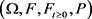﻿ 混合时滞随机Hopfield神经网络的均方渐近稳定性

# 混合时滞随机Hopfield神经网络的均方渐近稳定性Mean Square Asymptotic Stability of Stochastic Hopfield Neural Networks with Mixed Delays

Abstract: This paper considers a stochastic Hopfield neural network model with mixed delays, the mixed delays of the model are composed of constant fixed delay and continuously distributed delay. Li and Ding (2017) introduced this model and discussed its properties. In this paper, we will continue to study this model. Therefore, the main purpose of this paper is to obtain the criteria for the mean-square asymptotic stability of stochastic Hopfield neural networks with mixed delays through research and analysis. In addition, the methods we used are Lyapunov function method, Itô’s formula method and inequality method. First of all, we construct a suitable Lyapunov function. Then we apply Itô’s formula to the Lyapunov function. By calculation, we obtain the condition for judging the mean-square asymptotic stability of stochastic Hopfield neural networks with mixed delays. Lastly, we give an example to verify the results we obtained.

1. 引言

2. 模型，符号和假设

$\left\{\begin{array}{l}\text{d}{x}_{i}\left(t\right)=\left[-{c}_{i}{x}_{i}\left(t\right)+{\sum }_{j=1}^{n}{a}_{ij}{f}_{j}\left({x}_{j}\left(t\right)\right)+{\sum }_{j=1}^{n}{b}_{ij}{g}_{j}\left({x}_{j}\left(t-{\tau }_{j}\right)\right)\\ \text{\hspace{0.17em}}\text{\hspace{0.17em}}\text{\hspace{0.17em}}\text{\hspace{0.17em}}\text{\hspace{0.17em}}\text{\hspace{0.17em}}\text{\hspace{0.17em}}\text{\hspace{0.17em}}\text{\hspace{0.17em}}\text{\hspace{0.17em}}\text{\hspace{0.17em}}+{\sum }_{j=1}^{n}{d}_{ij}{\int }_{-\infty }^{t}{k}_{ij}\left(t-s\right){h}_{j}\left({x}_{j}\left(s\right)\right)\text{d}s\right]\text{d}t\\ \text{\hspace{0.17em}}\text{\hspace{0.17em}}\text{\hspace{0.17em}}\text{\hspace{0.17em}}\text{\hspace{0.17em}}\text{\hspace{0.17em}}\text{\hspace{0.17em}}\text{\hspace{0.17em}}\text{\hspace{0.17em}}\text{\hspace{0.17em}}\text{\hspace{0.17em}}+{\sum }_{j=1}^{n}{\sigma }_{ij}\left({x}_{j}\left(t\right),{x}_{j}\left(t-{\tau }_{j}\right),{\int }_{-\infty }^{t}{k}_{ij}\left(t-s\right){\phi }_{j}\left({x}_{j}\left(s\right)\right)\text{d}s\right)\text{d}{\omega }_{j}\left(t\right),\\ {x}_{i}\left(u\right)={\xi }_{i}\left(u\right),\text{\hspace{0.17em}}\text{\hspace{0.17em}}\text{\hspace{0.17em}}\text{\hspace{0.17em}}\text{\hspace{0.17em}}\text{\hspace{0.17em}}-\infty (1)

$\left\{\begin{array}{l}\text{d}x\left(t\right)=\left[-Cx\left(t\right)+Af\left(x\left(t\right)\right)+Bg\left(x\left(t-\tau \right)\right)+D{\int }_{-\infty }^{t}K\left(t-s\right)h\left(x\left(s\right)\right)\text{d}s\right]\text{d}t\\ \text{\hspace{0.17em}}\text{\hspace{0.17em}}\text{\hspace{0.17em}}\text{\hspace{0.17em}}\text{\hspace{0.17em}}\text{\hspace{0.17em}}\text{\hspace{0.17em}}\text{\hspace{0.17em}}\text{\hspace{0.17em}}\text{\hspace{0.17em}}\text{\hspace{0.17em}}+\sigma \left(x\left(t\right),x\left(t-\tau \right),{\int }_{-\infty }^{t}K\left(t-s\right)\phi \left(x\left(s\right)\right)\text{d}s\right)\text{d}\omega \left(t\right),\\ x\left(u\right)=\xi \left(u\right),\text{\hspace{0.17em}}\text{\hspace{0.17em}}\text{\hspace{0.17em}}\text{\hspace{0.17em}}\text{\hspace{0.17em}}\text{\hspace{0.17em}}-\infty (2)是一个具有滤子 ${\left\{{F}_{t}\right\}}_{t\ge 0}$ 且满足通常条件的完全概率空间，即，当 ${F}_{0}$ 包含所有P-空集时，它是右连续的和递增的。 $C\left(\left[-\tau ,0\right],{R}^{n}\right)$ 是从 $\left[-\tau ,0\right]$${R}^{n}$ 的连续函数族，其范数为 $‖\psi ‖={\mathrm{sup}}_{-\tau \le \theta \le 0}|\psi \left(\theta \right)|$ 并且 $\tau >0$${C}_{{F}_{0}}^{b}\left(\left[-\tau ,0\right],{R}^{n}\right)$ 定义了一族所有 ${F}_{0}$ 可测有界 $C\left(\left[-\tau ,0\right],{R}^{n}\right)$ 值随机变量$\omega \left(t\right)={\left({\omega }_{1}\left(t\right),\cdots ,{\omega }_{n}\left(t\right)\right)}^{\text{T}}$ 是n维布朗运动。对于 $i\ne j$${\omega }_{i}$${\omega }_{j}$ 互相独立，E是期望。

${C}^{2,1}\left[{R}^{n}×{R}^{+}\to {R}^{+}\right]$ 定义了一族 ${R}^{n}×{R}^{+}$ 上对x二次可微，对t一次可微的非负函数 $V\left(x,t\right)$。定义

$\begin{array}{c}LV\left(x\left(t\right),t\right)={V}_{t}\left(x,t\right)+{V}_{x}\left(x,t\right)\left[-Cx\left(t\right)+Af\left(x\left(t\right)\right)+Bg\left(x\left(t-\tau \right)\right)\\ \text{\hspace{0.17em}}\text{\hspace{0.17em}}+D{\int }_{-\infty }^{t}K\left(t-s\right)h\left(x\left(s\right)\right)\text{d}s\right]\text{d}t\\ \text{\hspace{0.17em}}\text{\hspace{0.17em}}+\frac{1}{2}\text{trace}\left[{\sigma }^{\text{T}}\left(x\left(t\right),x\left(t-\tau \right),{\int }_{-\infty }^{t}K\left(t-s\right)\phi \left(x\left(s\right)\right)\text{d}s\right)\\ \text{\hspace{0.17em}}\text{\hspace{0.17em}}×{V}_{xx}\left(x,t\right)\sigma \left(x\left(t\right),x\left(t-\tau \right),{\int }_{-\infty }^{t}K\left(t-s\right)\phi \left(x\left(s\right)\right)\text{d}s\right)\right],\end{array}$ (3)

2.1. 假设

a) 函数 ${f}_{j}\left(x\right)$${g}_{j}\left(x\right)$${h}_{j}\left(x\right)$${\phi }_{j}\left(x\right)$${\sigma }_{ij}\left(x,y,z\right)$ 满足：

${f}_{j}\left(0\right)={g}_{j}\left(0\right)={\phi }_{j}\left(0\right)=0$，且 ${\sigma }_{ij}\left(0,0,0\right)=0$$i,j=1,\cdots ,n$

b) 函数 ${f}_{j}\left(x\right)$${g}_{j}\left(x\right)$${h}_{j}\left(x\right)$${\phi }_{j}\left(x\right)$ 是利普希茨连续的，即，存在非负常数 ${L}_{j}$${M}_{j}$${N}_{j}$${U}_{j}$ 使得

$|{f}_{j}\left(x\right)-{f}_{j}\left(y\right)|\le {L}_{j}|x-y|,$ (4)

$|{g}_{j}\left(x\right)-{g}_{j}\left(y\right)|\le {M}_{j}|x-y|,$ (5)

$|{h}_{j}\left(x\right)-{h}_{j}\left(y\right)|\le {N}_{j}|x-y|,$ (6)

$|{\phi }_{j}\left(x\right)-{\phi }_{j}\left(y\right)|\le {U}_{j}|x-y|.$ (7)

c) 下面的不等式是成立的：(8)

2.2. 定义

3. 主要的结果

$\begin{array}{l}-2PC+PA{Q}^{-1}{A}^{\text{T}}P+LQL+PB{Q}^{-1}{B}^{\text{T}}P+{P}_{2}\\ +PD{Q}^{-1}{D}^{\text{T}}P+MQM+NQN+{P}_{1}+URU<0,\end{array}$

$\begin{array}{l}LV\left(x\left(t\right),t\right)\\ =2{x}^{\text{T}}\left(t\right)P\left[-Cx\left(t\right)+Af\left(x\left(t\right)\right)+Bg\left(x\left(t-\tau \right)\right)+D{\int }_{-\infty }^{t}K\left(t-s\right)h\left(x\left(s\right)\right)\text{d}s\right]\\ \text{\hspace{0.17em}}\text{\hspace{0.17em}}\text{ }\text{ }+{x}^{\text{T}}\left(t\right)\left({P}_{2}+MQM\right)x\left(t\right)-{x}^{\text{T}}\left(t-\tau \right)\left({P}_{2}+MQM\right)x\left(t-\tau \right)\\ \text{\hspace{0.17em}}\text{\hspace{0.17em}}\text{ }\text{ }+\underset{i=1}{\overset{n}{\sum }}\underset{j=1}{\overset{n}{\sum }}{q}_{j}{\int }_{0}^{\infty }{k}_{ij}\left(\eta \right){h}_{j}^{2}\left({x}_{j}\left(t\right)\right)\text{d}\eta -\underset{i=1}{\overset{n}{\sum }}\underset{j=1}{\overset{n}{\sum }}{q}_{j}{\int }_{0}^{\infty }{k}_{ij}\left(\eta \right){h}_{j}^{2}\left({x}_{j}\left(t-\eta \right)\right)\text{d}\eta \\ \text{\hspace{0.17em}}\text{\hspace{0.17em}}\text{ }\text{ }+\underset{i=1}{\overset{n}{\sum }}\underset{j=1}{\overset{n}{\sum }}{r}_{j}{\int }_{0}^{\infty }{k}_{ij}\left(\xi \right){\phi }_{j}^{2}\left({x}_{j}\left(t\right)\right)\text{d}\xi -\underset{i=1}{\overset{n}{\sum }}\underset{j=1}{\overset{n}{\sum }}{r}_{j}{\int }_{0}^{\infty }{k}_{ij}\left(\xi \right){\phi }_{j}^{2}\left({x}_{j}\left(t-\xi \right)\right)\text{d}\xi \end{array}$

$\begin{array}{l}\text{\hspace{0.17em}}\text{\hspace{0.17em}}\text{ }\text{ }+\text{trace}\left[{\sigma }^{\text{T}}\left(x\left(t\right),x\left(t-\tau \right),{\int }_{-\infty }^{t}K\left(t-s\right)\phi \left(x\left(s\right)\right)\text{d}s\right)\\ \text{\hspace{0.17em}}\text{\hspace{0.17em}}\text{ }\text{ }×P\sigma \left(x\left(t\right),x\left(t-\tau \right),{\int }_{-\infty }^{t}K\left(t-s\right)\phi \left(x\left(s\right)\right)\text{d}s\right)\right]\\ =-2{x}^{\text{T}}\left(t\right)PCx\left(t\right)+2{x}^{\text{T}}\left(t\right)PAf\left(x\left(t\right)\right)+2{x}^{\text{T}}\left(t\right)PBg\left(x\left(t-\tau \right)\right)\\ \text{\hspace{0.17em}}\text{\hspace{0.17em}}\text{ }\text{ }+2{x}^{\text{T}}PD{\int }_{-\infty }^{t}K\left(t-s\right)h\left(x\left(s\right)\right)\text{d}s+{h}^{\text{T}}\left(x\left(t\right)\right)Qh\left(x\left(t\right)\right)\\ \text{\hspace{0.17em}}\text{\hspace{0.17em}}\text{ }\text{ }+{x}^{\text{T}}\left(t\right)\left({P}_{2}+MQM\right)x\left(t\right)-{x}^{\text{T}}\left(t-\tau \right)\left({P}_{2}+MQM\right)x\left(t-\tau \right)\end{array}$$\begin{array}{l}\text{\hspace{0.17em}}\text{\hspace{0.17em}}\text{ }\text{ }+2{x}^{\text{T}}PD{\int }_{-\infty }^{t}K\left(t-s\right)h\left(x\left(s\right)\right)\text{d}s+{x}^{\text{T}}\left(t\right)\left({P}_{2}+MQM\right)x\left(t\right)\\ \text{\hspace{0.17em}}\text{\hspace{0.17em}}\text{ }\text{ }-{x}^{\text{T}}\left(t-\tau \right)\left({P}_{2}+MQM\right)x\left(t-\tau \right)+{h}^{\text{T}}\left(x\left(t\right)\right)Qh\left(x\left(t\right)\right)\\ \text{\hspace{0.17em}}\text{\hspace{0.17em}}\text{ }\text{ }-\underset{i=1}{\overset{n}{\sum }}\underset{j=1}{\overset{n}{\sum }}{q}_{j}{\int }_{0}^{\infty }{k}_{ij}\left(\eta \right){h}_{j}^{2}\left({x}_{j}\left(t-\eta \right)\right)\text{d}\eta +{\phi }^{\text{T}}\left(x\left(t\right)\right)R\phi \left(x\left(t\right)\right)\\ \text{\hspace{0.17em}}\text{\hspace{0.17em}}\text{ }\text{ }-\underset{i=1}{\overset{n}{\sum }}\underset{j=1}{\overset{n}{\sum }}{r}_{j}{\int }_{0}^{\infty }{k}_{ij}\left(\xi \right){\phi }_{j}^{2}\left({x}_{j}\left(t-\xi \right)\right)\text{d}\xi +{x}^{\text{T}}\left(t\right){P}_{1}x\left(t\right)+{x}^{\text{T}}\left(t-\tau \right){P}_{2}x\left(t-\tau \right)\\ \text{\hspace{0.17em}}\text{\hspace{0.17em}}\text{ }\text{ }+{\left({\int }_{-\infty }^{t}K\left(t-s\right)\phi \left(x\left(s\right)\right)\text{d}s\right)}^{\text{T}}R\left({\int }_{-\infty }^{t}K\left(t-s\right)\phi \left(x\left(s\right)\right)\text{d}s\right)\end{array}$

$\begin{array}{l}=-2{x}^{\text{T}}\left(t\right)PCx\left(t\right)+2{x}^{\text{T}}\left(t\right)PAf\left(x\left(t\right)\right)+2{x}^{\text{T}}\left(t\right)PBg\left(x\left(t-\tau \right)\right)\\ \text{\hspace{0.17em}}\text{\hspace{0.17em}}\text{ }\text{ }+2{x}^{\text{T}}PD{\int }_{-\infty }^{t}K\left(t-s\right)h\left(x\left(s\right)\right)\text{d}s+{x}^{\text{T}}\left(t\right)\left({P}_{2}+MQM\right)x\left(t\right)\\ \text{\hspace{0.17em}}\text{\hspace{0.17em}}\text{ }\text{ }-{x}^{\text{T}}\left(t-\tau \right)\left({P}_{2}+MQM\right)x\left(t-\tau \right)+{x}^{\text{T}}\left(t\right)NQNx\left(t\right)\\ \text{\hspace{0.17em}}\text{\hspace{0.17em}}\text{ }\text{ }-{\left({\int }_{-\infty }^{t}K\left(t-s\right)h\left(x\left(s\right)\right)\text{d}s\right)}^{\text{T}}Q\left({\int }_{-\infty }^{t}K\left(t-s\right)h\left(x\left(s\right)\right)\text{d}s\right)\\ \text{\hspace{0.17em}}\text{\hspace{0.17em}}\text{ }\text{ }-{\left({\int }_{-\infty }^{t}K\left(t-s\right)\phi \left(x\left(s\right)\right)\text{d}s\right)}^{\text{T}}R\left({\int }_{-\infty }^{t}K\left(t-s\right)\phi \left(x\left(s\right)\right)\text{d}s\right)\end{array}$

$\begin{array}{l}\text{\hspace{0.17em}}\text{\hspace{0.17em}}\text{ }\text{ }+{x}^{\text{T}}\left(t\right)URUx\left(t\right)+{x}^{\text{T}}\left(t\right){P}_{1}x\left(t\right)+{x}^{\text{T}}\left(t-\tau \right){P}_{2}x\left(t-\tau \right)\\ \text{\hspace{0.17em}}\text{\hspace{0.17em}}\text{ }\text{ }+{\left({\int }_{-\infty }^{t}K\left(t-s\right)\phi \left(x\left(s\right)\right)\text{d}s\right)}^{T}R\left({\int }_{-\infty }^{t}K\left(t-s\right)\phi \left(x\left(s\right)\right)\text{d}s\right)\end{array}$ (9)

$\begin{array}{c}2{x}^{\text{T}}\left(t\right)PAf\left(x\left(t\right)\right)\le {x}^{\text{T}}\left(t\right)PA{Q}^{-1}{A}^{\text{T}}{P}^{\text{T}}x\left(t\right)+{f}^{\text{T}}\left(x\left(t\right)\right)Qf\left(x\left(t\right)\right)\\ \le {x}^{\text{T}}\left(t\right)\left(PA{Q}^{-1}{A}^{\text{T}}P+LQL\right)x\left(t\right),\end{array}$ (10)

$\begin{array}{c}2{x}^{\text{T}}\left(t\right)PBg\left(x\left(t-\tau \right)\right)\le {x}^{\text{T}}\left(t\right)PB{Q}^{-1}{B}^{\text{T}}{P}^{\text{T}}x\left(t\right)+{g}^{\text{T}}\left(x\left(t-\tau \right)\right)Qg\left(x\left(t-\tau \right)\right)\\ \le {x}^{\text{T}}\left(t\right)\left(PB{Q}^{-1}{B}^{\text{T}}Px\left(t\right)+x\left(t-\tau \right)MQM\right)x\left(t-\tau \right),\end{array}$ (11)

$\begin{array}{l}2{x}^{\text{T}}\left(t\right)PD{\int }_{-\infty }^{t}K\left(t-s\right)h\left(x\left(s\right)\right)\text{d}s\\ \le {x}^{\text{T}}\left(t\right)PD{Q}^{-1}{D}^{\text{T}}Px\left(t\right)+{\left({\int }_{-\infty }^{t}K\left(t-s\right)h\left(x\left(s\right)\right)\text{d}s\right)}^{\text{T}}P\left({\int }_{-\infty }^{t}K\left(t-s\right)h\left(x\left(s\right)\right)\text{d}s\right).\end{array}$ (12)

$\begin{array}{c}LV\left(x\left(t\right),t\right)\le {x}^{\text{T}}\left(t\right)\left[-2PC+PA{Q}^{-1}{A}^{\text{T}}P+LQL+PB{Q}^{-1}{B}^{\text{T}}P+{P}_{2}\\ \text{\hspace{0.17em}}\text{\hspace{0.17em}}+PD{Q}^{-1}DP+MQM+NQN+{P}_{1}+URU\right]x\left(t\right)\end{array}$

4. 例子

$\begin{array}{l}\text{d}{x}_{1}\left(t\right)=\left[-{c}_{1}{x}_{1}\left(t\right)+{a}_{11}{f}_{1}\left({x}_{1}\left(t\right)\right)+{a}_{12}{f}_{2}\left({x}_{2}\left(t\right)\right)+{b}_{11}{g}_{1}\left({x}_{1}\left(t-{\tau }_{1}\right)\right)\\ \text{\hspace{0.17em}}\text{\hspace{0.17em}}\text{\hspace{0.17em}}\text{\hspace{0.17em}}\text{\hspace{0.17em}}\text{\hspace{0.17em}}\text{\hspace{0.17em}}\text{\hspace{0.17em}}\text{\hspace{0.17em}}\text{\hspace{0.17em}}\text{\hspace{0.17em}}+{b}_{12}{g}_{2}\left({x}_{2}\left(t-{\tau }_{2}\right)\right)+{d}_{11}{\int }_{-\infty }^{t}{k}_{11}\left(t-s\right){h}_{1}\left({x}_{1}\left(s\right)\right)\text{d}s\\ \text{\hspace{0.17em}}\text{\hspace{0.17em}}\text{\hspace{0.17em}}\text{\hspace{0.17em}}\text{\hspace{0.17em}}\text{\hspace{0.17em}}\text{\hspace{0.17em}}\text{\hspace{0.17em}}\text{\hspace{0.17em}}\text{\hspace{0.17em}}\text{\hspace{0.17em}}+{d}_{12}{\int }_{-\infty }^{t}{k}_{12}\left(t-s\right){h}_{2}\left({x}_{2}\left(s\right)\right)\text{d}s\right]\text{d}t\\ \text{\hspace{0.17em}}\text{\hspace{0.17em}}\text{\hspace{0.17em}}\text{\hspace{0.17em}}\text{\hspace{0.17em}}\text{\hspace{0.17em}}\text{\hspace{0.17em}}\text{\hspace{0.17em}}\text{\hspace{0.17em}}\text{\hspace{0.17em}}\text{\hspace{0.17em}}+{\sigma }_{11}\left({x}_{1}\left(t\right),{x}_{1}\left(t-{\tau }_{1}\right),{\int }_{-\infty }^{t}{k}_{11}\left(t-s\right){\phi }_{1}\left({x}_{1}\left(s\right)\right)\text{d}s\right)\text{d}{\omega }_{1}\left(t\right)\\ \text{\hspace{0.17em}}\text{\hspace{0.17em}}\text{\hspace{0.17em}}\text{\hspace{0.17em}}\text{\hspace{0.17em}}\text{\hspace{0.17em}}\text{\hspace{0.17em}}\text{\hspace{0.17em}}\text{\hspace{0.17em}}\text{\hspace{0.17em}}\text{\hspace{0.17em}}+{\sigma }_{12}\left({x}_{2}\left(t\right),{x}_{2}\left(t-{\tau }_{2}\right),{\int }_{-\infty }^{t}{k}_{12}\left(t-s\right){\phi }_{2}\left({x}_{2}\left(s\right)\right)\text{d}s\right)\text{d}{\omega }_{2}\left(t\right),\end{array}$

$\begin{array}{l}\text{d}{x}_{2}\left(t\right)=\left[-{c}_{2}{x}_{2}\left(t\right)+{a}_{21}{f}_{1}\left({x}_{1}\left(t\right)\right)+{a}_{22}{f}_{2}\left({x}_{2}\left(t\right)\right)+{b}_{21}{g}_{1}\left({x}_{1}\left(t-{\tau }_{1}\right)\right)\\ \text{\hspace{0.17em}}\text{\hspace{0.17em}}\text{\hspace{0.17em}}\text{\hspace{0.17em}}\text{\hspace{0.17em}}\text{\hspace{0.17em}}\text{\hspace{0.17em}}\text{\hspace{0.17em}}\text{\hspace{0.17em}}\text{\hspace{0.17em}}\text{\hspace{0.17em}}\text{\hspace{0.17em}}+{b}_{22}{g}_{2}\left({x}_{2}\left(t-{\tau }_{2}\right)\right)+{d}_{21}{\int }_{-\infty }^{t}{k}_{21}\left(t-s\right){h}_{1}\left({x}_{1}\left(s\right)\right)\text{d}s\\ \text{\hspace{0.17em}}\text{\hspace{0.17em}}\text{\hspace{0.17em}}\text{\hspace{0.17em}}\text{\hspace{0.17em}}\text{\hspace{0.17em}}\text{\hspace{0.17em}}\text{\hspace{0.17em}}\text{\hspace{0.17em}}\text{\hspace{0.17em}}\text{\hspace{0.17em}}\text{\hspace{0.17em}}+{d}_{22}{\int }_{-\infty }^{t}{k}_{22}\left(t-s\right){h}_{2}\left({x}_{2}\left(s\right)\right)\text{d}s\right]\text{d}t\\ \text{\hspace{0.17em}}\text{\hspace{0.17em}}\text{\hspace{0.17em}}\text{\hspace{0.17em}}\text{\hspace{0.17em}}\text{\hspace{0.17em}}\text{\hspace{0.17em}}\text{\hspace{0.17em}}\text{\hspace{0.17em}}\text{\hspace{0.17em}}\text{\hspace{0.17em}}\text{\hspace{0.17em}}+{\sigma }_{21}\left({x}_{1}\left(t\right),{x}_{1}\left(t-{\tau }_{1}\right),{\int }_{-\infty }^{t}{k}_{21}\left(t-s\right){\phi }_{1}\left({x}_{1}\left(s\right)\right)\text{d}s\right)\text{d}{\omega }_{1}\left(t\right)\\ \text{\hspace{0.17em}}\text{\hspace{0.17em}}\text{\hspace{0.17em}}\text{\hspace{0.17em}}\text{\hspace{0.17em}}\text{\hspace{0.17em}}\text{\hspace{0.17em}}\text{\hspace{0.17em}}\text{\hspace{0.17em}}\text{\hspace{0.17em}}\text{\hspace{0.17em}}\text{\hspace{0.17em}}+{\sigma }_{22}\left({x}_{2}\left(t\right),{x}_{2}\left(t-{\tau }_{2}\right),{\int }_{-\infty }^{t}{k}_{22}\left(t-s\right){\phi }_{2}\left({x}_{2}\left(s\right)\right)\text{d}s\right)\text{d}{\omega }_{2}\left(t\right),\end{array}$ (13)

$C=\left(\begin{array}{cc}{c}_{11}& 0\\ 0& {c}_{22}\end{array}\right)=\left(\begin{array}{cc}6& 0\\ 0& 8\end{array}\right),\text{\hspace{0.17em}}\text{\hspace{0.17em}}A=\left(\begin{array}{cc}{a}_{11}& {a}_{12}\\ {a}_{21}& {a}_{22}\end{array}\right)=\left(\begin{array}{cc}0.2& 0.1\\ 0.3& 0.4\end{array}\right),$

$B=\left(\begin{array}{cc}{b}_{11}& {b}_{12}\\ {b}_{21}& {b}_{22}\end{array}\right)=\left(\begin{array}{cc}0.4& 0.1\\ 0.2& 0.2\end{array}\right),\text{\hspace{0.17em}}\text{\hspace{0.17em}}D=\left(\begin{array}{cc}{d}_{11}& {d}_{12}\\ {d}_{21}& {d}_{22}\end{array}\right)=\left(\begin{array}{cc}0.1& 0.2\\ 0.4& 0.3\end{array}\right).$$\begin{array}{l}{\sigma }_{12}\left({x}_{2}\left(t\right),{x}_{2}\left(t-{\tau }_{2}\right),{\int }_{-\infty }^{t}{k}_{12}\left(t-s\right){\phi }_{2}\left({x}_{2}\left(s\right)\right)\text{d}s\right)\\ =\frac{{x}_{2}\left(t\right)}{4}+\frac{{x}_{2}\left(t-{\tau }_{2}\right)}{2}+\frac{1}{2}{\int }_{-\infty }^{t}{k}_{12}\left(t-s\right){\phi }_{2}\left({x}_{2}\left(s\right)\right)\text{d}s,\end{array}$

$\begin{array}{l}{\sigma }_{21}\left({x}_{1}\left(t\right),{x}_{1}\left(t-{\tau }_{1}\right),{\int }_{-\infty }^{t}{k}_{21}\left(t-s\right){\phi }_{1}\left({x}_{1}\left(s\right)\right)\text{d}s\right)\\ =\frac{{x}_{1}\left(t\right)}{2}+\frac{{x}_{1}\left(t-{\tau }_{1}\right)}{4}+\frac{1}{2}{\int }_{-\infty }^{t}{k}_{21}\left(t-s\right){\phi }_{1}\left({x}_{1}\left(s\right)\right)\text{d}s,\end{array}$

$\begin{array}{l}{\sigma }_{22}\left({x}_{2}\left(t\right),{x}_{2}\left(t-{\tau }_{2}\right),{\int }_{-\infty }^{t}{k}_{22}\left(t-s\right){\phi }_{2}\left({x}_{2}\left(s\right)\right)\text{d}s\right)\\ =\frac{{x}_{1}\left(t\right)}{2}+\frac{{x}_{1}\left(t-{\tau }_{1}\right)}{2}+\frac{1}{4}{\int }_{-\infty }^{t}{k}_{22}\left(t-s\right){\phi }_{2}\left({x}_{2}\left(s\right)\right)\text{d}s.\end{array}$

${f}_{j}\left(x\right)={g}_{j}\left(x\right)={h}_{j}\left(x\right)={\phi }_{j}\left(x\right)=\mathrm{tanh}\left(x\right)$${k}_{ij}\left(t\right)={\text{e}}^{-t}$$i,j=1,2$${\tau }_{1}={\tau }_{2}=1$，然后我们有 ${L}_{1}={L}_{2}=1$${M}_{1}={M}_{2}=1$${N}_{1}={N}_{2}=1$${U}_{1}={U}_{2}=1$

$\begin{array}{l}-2PC+PA{Q}^{-1}{A}^{\text{T}}P+LQL+PB{Q}^{-1}{B}^{\text{T}}P+{P}_{2}\\ +PD{Q}^{-1}{D}^{\text{T}}P+MQM+NQN+{P}_{1}+URU=\left(\begin{array}{cc}-7.43& 0.3\\ 0.3& -11.02\end{array}\right)<0,\end{array}$

5. 结论

 Hopfield, J. (1982) Neural Networks and Physical Systems with Emergent Collective Computational Abilities. Proceedings of the National Academy of Sciences, 79, 2554-2558.
https://doi.org/10.1073/pnas.79.8.2554

 郭鹏, 韩璞. Hopfield网络在优化计算中的应用[J]. 计算机仿真, 2002, 19(3): 37-39.

 韩琦. 神经网络的稳定性及其在联想记忆中的应用研究[D]: [博士学位论文]. 重庆: 重庆大学计算机学院, 2012.

 Shitonga, W., Duana, F., Mina, X. and Dewenc, H. (2007) Advanced Fuzzy Cellular Neural Networks: Application to CT Liver Images. Artificial Intelligence in Medicine, 39, 65-77.
https://doi.org/10.1016/j.artmed.2006.08.001

 Arik, S. (2000) Global Asymptotic Stability of a Class of Dynamical Neural Networks. IEEE Transactions on Circuits and Systems—I: Fundamental Theory and Applications, 47, 568-571.
https://doi.org/10.1109/81.841858

 徐晓惠, 张继业, 施继忠, 等. 脉冲干扰时滞复值神经网络的稳定性分析[J]. 哈尔滨工业大学学报, 2016, 48(3): 166-170.

 Bai, J., Lu, R., Xue, A., et al. (2015) Finite-Time Stability Analysis of Discrete-Time Fuzzy Hopfield Neural Network. Neurocomputing, 159, 263-267.
https://doi.org/10.1016/j.neucom.2015.01.051

 Xu, B., Liu, X. and Liao, X. (2006) Global Exponential Stability of High Order Hopfield Type Neural Networks. Applied Mathematics and Computation, 174, 98-116.
https://doi.org/10.1016/j.amc.2005.03.020

 Zhu, Q. and Cao, J. (2014) Mean-Square Exponential Input-to-state Stability of Stochastic Delayed Neural Networks. Neurocomputing, 131, 157-163.
https://doi.org/10.1016/j.neucom.2013.10.029

 Haykin, S. (1994) Neural Netw. Prentice-Hall, Upper Saddle Riv-er.

 Liao, X. and Mao, X. (1996) Exponential Stability and Instability of Stochastic Neural Networks. Stochastic Analysis and Applications, 14, 165-185.
https://doi.org/10.1080/07362999608809432

 Wan, L. and Sun, J. (2005) Mean Square Expo-nential Stability of Stochastic Delayed Hopfield Neural Networks. Physics Letters A, 343, 306-318.
https://doi.org/10.1016/j.physleta.2005.06.024

 Zhou, Q. and Wan, L. (2008) Exponential Stability of Stochastic Delayed Hopfield Neural Networks. Applied Mathematics and Computation, 199, 84-89.
https://doi.org/10.1016/j.amc.2007.09.025

 Chen, W. and Lu, X. (2013) Mean Square Exponential Stability of Uncertain Stochastic Delayed Neural Networks. Physics Letters A, 372, 1061-1069.
https://doi.org/10.1016/j.physleta.2007.09.009

 Guo, Y. (2009) Mean Square Global Asymptotic Stability of Stochastic Recurrent Neural Networks with Distributed Delays. Applied Mathematics and Computation, 215, 791-795.
https://doi.org/10.1016/j.amc.2009.06.002

 Ma, L. and Da, F. (2009) Mean-Square Exponential Stability of Stochastic Hopfield Neural Networks with Time-Varying Discrete and Distributed Delays. Physics Letters A, 373, 2154-2161.
https://doi.org/10.1016/j.physleta.2009.04.031

 Li, X. and Deng, D. (2017) Mean Square Exponential Stability of Stochastic Hopfield Neural Networks with Mixed Delays. Statistics & Probability Letters, 126, 88-96.
https://doi.org/10.1016/j.spl.2017.02.029

 Li, X., Deng, D. and Di, S. (2018) Exponential Stability of Stochastic Cellular Neural Networks with Mixed Delays. Communications in Statistics—Theory and Methods, 47, 4881-4894.

Top